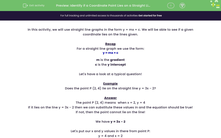# Identify if a Coordinate Point Lies on a Straight Line of Equation y = mx + c

In this worksheet, students will work out if a given coordinate lies on a given straight line graph.Key stage:  KS 3

Curriculum topic:   Algebra

Curriculum subtopic:   Use Coordinates in All Four Quadrants

Difficulty level:#### Worksheet Overview

In this activity, we will use straight line graphs in the form y = mx + c. We will be able to see if a given coordinate lies on the lines given.

Recap

For a straight line graph we use the form:

## y = mx + c

c is the y intercept

Let's have a look at a typical question!

Example

Does the point P (2, 4) lie on the straight line y = 3x - 2?

The point P (2, 4) means  when x = 2, y = 4

If it lies on the line y = 3x - 2 then we can substitute these values in and the equation should be true!

If not, then the point cannot lie on the line!

We have y = 3x - 2

Let's put our x and y values in there from point P:

y = 4 and x = 2

4 = (3x2) - 2

4 = 6 - 2

4 = 4  it's correct!

Therefore, the point P  does lie on the line y = 3x - 2

If this had not been correct, then the point P would not lie on the line!

Let's try some questions.### What is EdPlace?

We're your National Curriculum aligned online education content provider helping each child succeed in English, maths and science from year 1 to GCSE. With an EdPlace account you’ll be able to track and measure progress, helping each child achieve their best. We build confidence and attainment by personalising each child’s learning at a level that suits them.

Get started